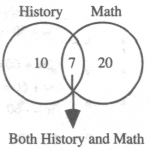## UCAT test tactics and preparation part 5: Counting Problems in UCAT1 year ago by Robert

## Counting problems in UCAT: an essential summary.

Your UCAT questions explained by the team at MedEntry as part of our new section UCAT test tactics and preparation.

### They may seem simple on paper, but counting problems contained within the UCAT exam can often cause much confusion among students. If you can understand the basic principles of counting problems and recognize when to apply them, you will be able to master many of the typical counting problem question types presented in the UCAT exam.

When counting elements that are in overlapping sets, the total number will equal the number in one group plus the number in the other group minus the number common to both groups. Venn diagrams are very helpful with these typical UCAT style problems.

Sample UCAT style question: If in a certain school 20 students are taking math and 10 are taking history and 7 are taking both, how many students are taking either math or history?(A) 23

(B) 25

(C) 29

(D) 37

By the principle stated above, we add 10 and 20 and then subtract 7 from the result.
Thus, there are (10 + 20) – 7 = 23 students. The answer is (B).

The number of integers between two integers inclusive is one more than their difference.

Sample UCAT style question: How many integers are there between 49 and 101, inclusive?

(A) 50

(B) 51

(C) 52

(D) 53

By the principle stated above, the number of integers between 49 and 101 inclusive is (101 – 49) + 1 = 53. The answer is (D). To make this clearer, choose smaller numbers, say, 9 and 11. The difference between 9 and 11 is 2. But there are three numbers between them inclusive—9, 10, and 11—one more than their difference.

Fundamental Principle of Counting: If an event occurs m times, and each of the m events is followed by a second event which occurs k times, then the first event follows the second event m * k times.

Sample UCAT style question: The following diagram illustrates the fundamental principle of counting for an event that occurs 3 times with each occurrence being followed by a second event that occurs 2 times for a total of 3 * 2 = 6 events:Sample UCAT style question: A drum contains 3 to 5 jars each of which contains 30 to 40 marbles. If 10 percent of the marbles are flawed, what is the greatest possible number of flawed marbles in the drum?

(A) 51

(B) 40

(C) 30

(D) 20

There are at most 5 jars each of which contains at most 40 marbles; so by the fundamental counting principle, there is at most 5 * 40 = 200 marbles in the drum. Since 10 percent of the marbles are flawed, there is at most 10% * 200 = 20 flawed marbles. The answer is (D).

For a more extensive data-base of UCAT questions and more detailed UCAT advice see MedEntry UCAT preparation.